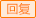84785040| 2022 11/26 20:27| 94人阅读xu老师

2022 11/26 20:48xu老师2022 11/26 20:57
（1）收入总额=800+40+60=900（万元） （2）职工福利费扣除限额=100*14%=14（万元），实际发生15万元，准予扣除14万元；职工教育经费扣除限额=100*8%=8（万元），实际发生1.5万元，准予扣除1.5万元；工会经费扣除限额=100*2.5%=2.5（万元），实际发生2万元，准予扣除2万元；诉讼费、违约金和逾期利息准予扣除，扣除金额合计=3+5+6=14（万元）；滞纳金4万元不准予扣除；准予扣除金额合计=100+14+1.5+2+14=131.5（万元） （3）不予扣除金额合计=1+4=5（万元） （4）准予扣除原材料损失金额=10+1.3-6=5.3（万元）